# Area of square

Calculate the content area of the square whose perimeter is 24 dm.

Result

S =  36 dm2

#### Solution:Leave us a comment of example and its solution (i.e. if it is still somewhat unclear...):

Showing 0 comments:Be the first to comment!## Next similar examples:

1. Square plate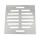From a square metal plate with a side long 6dm were cut two identical rectangular openings with dimensions of 2.5 dm and 2 dm. Calculate the remainder of the square plate.
2. SquaresCalculate the area of square and result round to square decimeters. a) a = 1,52 dm b) a = 13 268mm c) a = 562 cm d) a = 1,52 m
3. Perimeter from areaWhat is the perimeter of the square if its content is 64 cm2?
4. Perimeter of squareThe square has a circumference 17cm. What is its area?
5. Unknown number 24I think the number: a - is the same as the square area that has the 12th circumfence. What is this number? b - its half is 7 times bigger than its quarter. Is this the number?
6. Fence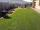Square garden has an area of 537 m2. How many meters netting is necessary to fence the garden?
7. Rectangle vs square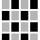The rectangle has dimensions of 13 × 10, square 8 × 8. Which shape has more area and how much above?
8. Carpet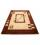What is the price of square carpet side length 3.25 m if one meter square cost 230 CZK?
9. RectangleThe rectangle area is 182 dm2, its base is 14 dm. How long is the other side? Calculate its perimeter.
10. Strip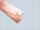From 5.9 cm wide strip should be cut rhombus with area 28 cm2. How long will be its side?
11. Garden plotCalculate how many meters of fence need to fence the square garden with length and width of 22 meters.
12. RectangleCalculate perimeter of the rectangle with sides a=2.4 m and b=1.9 m.
13. Addition of Roman numbersAdded together and write as decimal number: LXVII + MLXIV
14. CagesHonza had three cages (black, silver, gold) and three animals (guinea pig, rat and puppy). There was one animal in each cage. The golden cage stood to the left of the black cage. The silver cage stood on the right of the guinea pig cage. The rat was in the
15. CakesOn the bowl were a few cakes. Jane ate one-third of them, Dana ate a quarter of those cakes that remained. a) What part (of the original number of cakes) Dana ate? b) At least how many cakes could be (initially) on thebowl?
16. Math classificationIn 3A class are 27 students. One-third got a B in math and the rest got A. How many students received a B in math?
17. Foot in busIt was 102 people on the bus. 28 girls had two dogs. A 11 girls had one dog. At the next stop seceded 5 dogs (even with their owners). They got two boys together with three dogs. The bus drove one driver. How many foot were in bus?